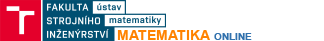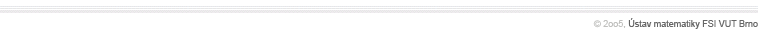Ordinary differential equationsBasic definitions, solution to a differential equation, general, particular, and singular solutions, Cauchy initial problemDifferential equations with separable variablesMethods for solving ordinary differential equations with separable variablesLinear differential equations with constant coefficientsIndependent solutions, Wronskian of a solution, properties of Wronskian, homogeneous and non-homogeneous problems, variation-of-constants method for finding a particular solution, special forms of right-hand-sidesMatrix functionsMethods of defining the value of a function at a square matrixSystems of linear differential equations with constant coefficientsHomogeneous and non-homogeneous systems of linear differential equations, fundamental solution matrix, solving systems of linear differential equations with constant coefficients using the exp(xA) matrix function, variation-of-constants method